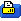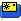This thread is privately moderated by cmdl, who may elect to delete unwanted replies.
Dec 20, 2019, 05:14 PM
http://www.sgvhumane.org/
Mini-HowTo

# Climate change - two simple steps

Source: https://archive.epa.gov/climatechang...sics/past.html

Step 1: Eyeballing (or use ruler) temperature change with respect to CO2 on the left two graphs (upper is CO2, lower is temperature). Total temp change (F) divided by Total CO2 change (ppm) = Rate of change of temp w.r.t. CO2.
Calculate the rate for the two left graphs (before industrialization) and the two right graphs (after industrialization).

Step 2: Compare the rates.
For the left graphs (before industrialization), I got rate of change = (-15 F to 5F) / (175ppm to 300ppm) = 20 F / 125 ppm = 0.16 F/ppm.
For the right graphs (after industrialization), I got rate of change = (-1 F to 1.5F) / (300ppm to 400ppm) = 2.5 F / 100 ppm = 0.025 F/ppm.

The rate of change of temperature w.r.t CO2 before industrialization is 0.16/0.025 = 6.4 times greater than after industrialization.
 Sign up now to remove ads between postsDec 21, 2019, 07:28 PM "Yo, that's a thermal" This is a problem related to climate sensitivity, and not simple rates of change. CO2 is a climate forcing; the relationship between CO2 and radiative forcing is logarithmic. There is much debate about the exact value of sensitivity, but even the skeptics would disagree with your analysis. When we talk about direct temperature changes in the climate, we use (degrees C) / (flux), where the units are C and W/m^2. CO2 levels will make the flux vary, both coming into the atmosphere, and what is being absorbed after emission off the Earth's surface. This will then effect the temperature of the troposphere. There is a "middleman" between CO2 and temperature (radiative forcing). The CO2 levels themselves will alter the rate of change of temperature w.r.t. them. https://scied.ucar.edu/climate-sensitivity-calculator Last edited by DJO; Dec 21, 2019 at 07:59 PM. Reason: clarity
 Dec 21, 2019, 07:35 PM "Yo, that's a thermal" The exact logarithmic formula is R = 5.35 * ln(C / C_0) R = radiative forcing (flux) in W/m^2 C = CO2 concentration in ppm C_0 = reference concetration, normally 280ppm (for before the industrial age). With C = 380 ppm and C_0 = 280 ppm, R = 1.633 With C = 410 ppm and C_0 = 280 ppm, R = 2.040 With C = 600 ppm and C_0 = 280 ppm, R = 4.077
 Dec 21, 2019, 07:37 PM "Yo, that's a thermal" More info if you are interested: https://skepticalscience.com/C02-emi...re-growth.html

 Thread ToolsShow Printable VersionEmail this Page